﻿ 采用封闭式加热炉同时生产海绵铁，石灰和无氮型煤气技术

# 采用封闭式加热炉同时生产海绵铁，石灰和无氮型煤气技术The Technology of Producing Sponge Iron, Lime and Nitrogen-Free Gas Simultaneously with Enclosed Heating Furnace

Abstract: In this paper, the theoretical and experimental results of simultaneous production of sponge iron, lime and nitrogen-free high calorific value gas by enclosed heating furnace are expounded. The oxygen in iron oxide is used to produce nitrogen-free gas directly. Nitrogen-free gas is produced from carbon dioxide decomposed by limestone and carbon, making use of lime to capture carbon dioxide in flue gas. The advantage of this technology is that natural resources are fully utilized. Coal consumption is greatly reduced and economic benefits are remarkable. Carbon dioxide emissions far exceed the IEA’s requirement to capture buried amounts. Various furnace types for producing sponge iron are discussed. Blast furnace type should be the least energy consumption furnace type. It also refers to “over capture”.

1. 引言

2. 碳还原氧化铁反应罐内的反应

$\text{C}+{\text{CO}}_{\text{2}}=\text{2CO}\text{\hspace{0.17em}}\text{\hspace{0.17em}}\text{\hspace{0.17em}}\Delta \text{H}=+\text{41}.\text{21}\text{\hspace{0.17em}}\text{kcal}/\text{mol}\left(+\text{172}.\text{538}\text{\hspace{0.17em}}\text{kj}/\text{mol}\right)$ (1)

${\text{3Fe}}_{\text{2}}{\text{O}}_{\text{3}}+\text{CO}={\text{2Fe}}_{\text{3}}{\text{O}}_{\text{4}}+{\text{CO}}_{\text{2}}$ (2)

${\text{Fe}}_{\text{3}}{\text{O}}_{\text{4}}+\text{CO}=\text{3FeO}+{\text{CO}}_{\text{2}}$ (3)

$\text{FeO}+\text{CO}=\text{Fe}+{\text{CO}}_{\text{2}}$ (4)

$\begin{array}{l}\text{\hspace{0.17em}}\text{\hspace{0.17em}}\text{\hspace{0.17em}}\text{\hspace{0.17em}}\text{\hspace{0.17em}}\text{\hspace{0.17em}}\text{\hspace{0.17em}}\text{2CO}←{\text{CO}}_{\text{2}}+\text{C}发生在碳粉层内\\ \text{\hspace{0.17em}}\text{\hspace{0.17em}}\text{\hspace{0.17em}}\text{\hspace{0.17em}}\text{\hspace{0.17em}}\text{\hspace{0.17em}}\text{\hspace{0.17em}}\text{\hspace{0.17em}}\text{\hspace{0.17em}}↓\text{\hspace{0.17em}}\text{\hspace{0.17em}}\text{\hspace{0.17em}}\text{\hspace{0.17em}}\text{\hspace{0.17em}}\text{\hspace{0.17em}}\text{\hspace{0.17em}}\text{\hspace{0.17em}}\text{\hspace{0.17em}}\text{\hspace{0.17em}}\text{\hspace{0.17em}}\text{\hspace{0.17em}}↑\\ \text{FeO}+\text{CO}\to \text{Fe}+{\text{CO}}_{\text{2}}发生在氧化铁粉层内\end{array}$

3. 两个实验测定

3.1. 煤气隧道窑中碳还原氧化铁过程测定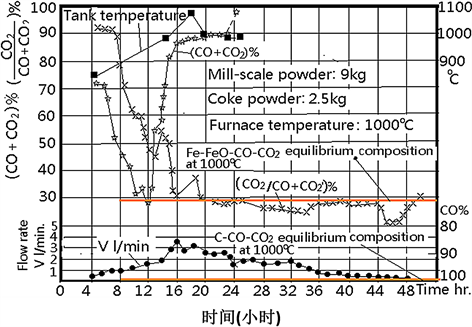Figure 1. 38.5 meters long tunnel kiln gas composition and flow rate in the reduction reaction tank (two red lines in the figure are $\text{FeO}+\text{CO}=\text{Fe}+{\text{CO}}_{\text{2}}$ and ${\text{CO}}_{\text{2}}+\text{C}+\text{2CO}$ reaction equilibrium composition lines, respectively)

1) 氧化铁还原反应是分阶段进行，即Fe2O3→Fe3O4→FeO→Fe。其中，FeO (浮氏体)还原时间延续最长，接近30小时。这个结果与教科书上描述的完全一致，与Fe-C-O反应平衡图或Fe-O相图是一致的。

2) 将测定的气体成分和碳气化反应以及FeO还原反应的平衡气相成分相比较，证明碳气化反应是还原过程反应速度最慢的环节，它控制了整个还原过程速度。因此，在还原剂碳中加入催化剂就能有效的加速还原过程速度，提高生产率。

3) 还原过程与渗碳过程截然分开。还原过程和渗碳过程之间存在一个“还原终点”，只有还原过程完全结束，FeO相完全消失以后气相成分才能转变为渗碳气氛。1000℃时，还原FeO的平衡气相成分中CO2含量约是28%，而渗碳时，与Fe3C相相平衡的CO2成分几乎为零，一氧化碳的含量几乎是100%。每天检查海绵铁锭质量时，只要海绵铁锭内有微量黑色氧化铁，碳的含量始终低于0.03%，表明没有进入渗碳阶段。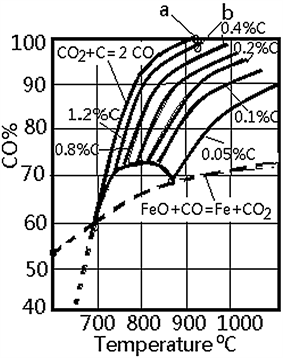Figure 2. Fe-CO-CO2 equilibrium diagram

3.2. 双料盒实验研究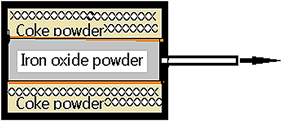Figure 3. Experimental box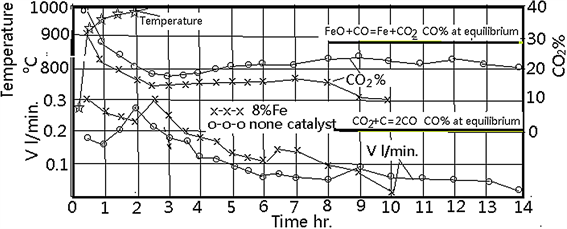Figure 4. Fe catalytic activityFigure 5. Fe-Cu catalytic activity

4. 利用石灰捕捉二氧化碳

${\text{CaCO}}_{\text{3}}=\text{CaO}+{\text{CO}}_{\text{2}}\text{\hspace{0.17em}}\text{\hspace{0.17em}}\text{\hspace{0.17em}}\Delta {\text{H}}_{\text{298k}}^{0}=\text{178}\text{\hspace{0.17em}}\text{kj}/\text{mol}$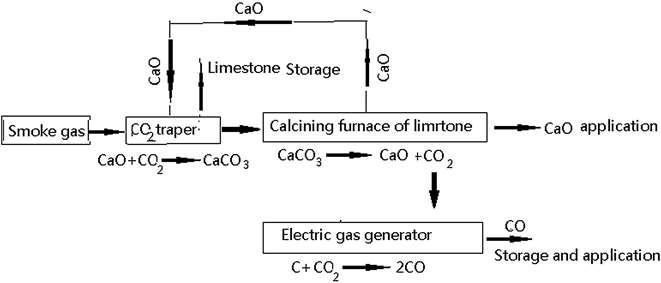Figure 6. Flow chart of lime capture carbon dioxide

5. 贮能指数与能耗

$\begin{array}{l}\text{C}+{\text{CO}}_{\text{2}}=\text{2CO}\text{\hspace{0.17em}}\text{\hspace{0.17em}}\text{\hspace{0.17em}}\text{\hspace{0.17em}}\text{\hspace{0.17em}}\text{\hspace{0.17em}}\text{\hspace{0.17em}}\text{\hspace{0.17em}}\text{\hspace{0.17em}}\text{\hspace{0.17em}}\text{\hspace{0.17em}}\text{\hspace{0.17em}}\text{\hspace{0.17em}}\text{\hspace{0.17em}}\text{\hspace{0.17em}}\text{\hspace{0.17em}}\text{\hspace{0.17em}}\text{\hspace{0.17em}}\text{\hspace{0.17em}}\text{\hspace{0.17em}}\text{ZN}=\text{3}.\text{52}\\ {\text{H}}_{\text{2}}\text{O}+\text{C}={\text{H}}_{\text{2}}+\text{CO}\text{\hspace{0.17em}}\text{\hspace{0.17em}}\text{\hspace{0.17em}}\text{\hspace{0.17em}}\text{\hspace{0.17em}}\text{\hspace{0.17em}}\text{\hspace{0.17em}}\text{\hspace{0.17em}}\text{\hspace{0.17em}}\text{\hspace{0.17em}}\text{\hspace{0.17em}}\text{\hspace{0.17em}}\text{\hspace{0.17em}}\text{\hspace{0.17em}}\text{ZN}=\text{4}.\text{35}\\ {\text{Fe}}_{\text{2}}{\text{O}}_{\text{3}}+\text{3C}=\text{2Fe}+\text{3CO}\text{\hspace{0.17em}}\text{\hspace{0.17em}}\text{\hspace{0.17em}}\text{\hspace{0.17em}}\text{\hspace{0.17em}}\text{\hspace{0.17em}}\text{\hspace{0.17em}}\text{\hspace{0.17em}}\text{ZN}=\text{1}.\text{78}\\ {\text{CaCO}}_{\text{3}}+\text{2C}=\text{CaO}+\text{2CO}\text{\hspace{0.17em}}\text{\hspace{0.17em}}\text{\hspace{0.17em}}\text{\hspace{0.17em}}\text{\hspace{0.17em}}\text{ZN}=\text{1}.\text{68}\end{array}$

6. 炉型选择，技术与产品

7. 结束语

1) 火力发电厂是耗煤大户，也是大量排放二氧化碳的用户，估计其排放量是水泥钢铁企业产生的三倍以上，是必须捕捉二氧化碳的主要企业。

2) 柴禾塑料垃圾焚烧炉应该是大量排放二氧化碳的另一个大户。一吨PE或PP型塑料，根据结构式计算，完全燃烧后可以产生3.14吨二氧化碳(一吨碳完全燃烧后产生3.67吨二氧化碳)。但是与二氧化碳反应后能够生产4800立米煤气。

3) 水泥，钢铁生产排放的二氧化碳数量约为全球总排放量330亿吨(2017年)的10%，几乎达到了联合国能源署要求年捕捉34亿吨二氧化碳的要求。可喜的是这两个生产项目捕捉二氧化碳非常简单，只要在石灰窑或还原反应罐中多加一点碳就可以了，碳的加入量可以根据化学反应式计算得到。

 金家敏. 铁鳞还原过程的研究和提高铁粉产量、质量的措施[J]. 机械工程材料, 1977(3): 38-42.

 金家敏. 铁粉生产10年[Z]. 萧山: 中国华东区粉末冶金会议资料, 1977.

 金家敏. 还原铁粉生产的合理工艺流程[Z]. 上海粉末冶金厂资料室, 1980.

 金家敏. 江国源, 任家瑛 朱传征. (1982) 碳中矿物杂质对碳还原氧化铁过程的催化与毒化作用机理——电子循环授受助催化理论验证文章之一[C]. 昆明, 全国粉末冶金会议资料.

 Jin, J.M. and Jiang, G.Y. (1988) The Diverse Effects of Various Mineral Impurities on the Carbon Gasification Reaction. Proceedings of International Powder Metallurgy Conference.

 金家敏. 利用捕捉的二氧化碳,煤炭和柴禾生产煤气——[利用捕捉的二氧化碳贮能减排]一文的补充[J]. 低碳经济, 2019, 8(4): 5.

 Jin, J.M. (2020) Making use of CO2 Capture for Saving Earth and Human—A Comparison of “Carbon Capture and Storage—CCS” and “Carbon Capture and Storage Energy—CCSE”. Global Journal of Human-Social Science Research, 20, 17-20.
https://doi.org/10.34257/GJHSSHVOL20IS2PG17

Top